# 1467 Challenge Puzzle

### Challenge Puzzle:

Use logic to solve this Challenge Puzzle. The given clues work together to make finding the unique solution a little easier than usual. Have fun with it!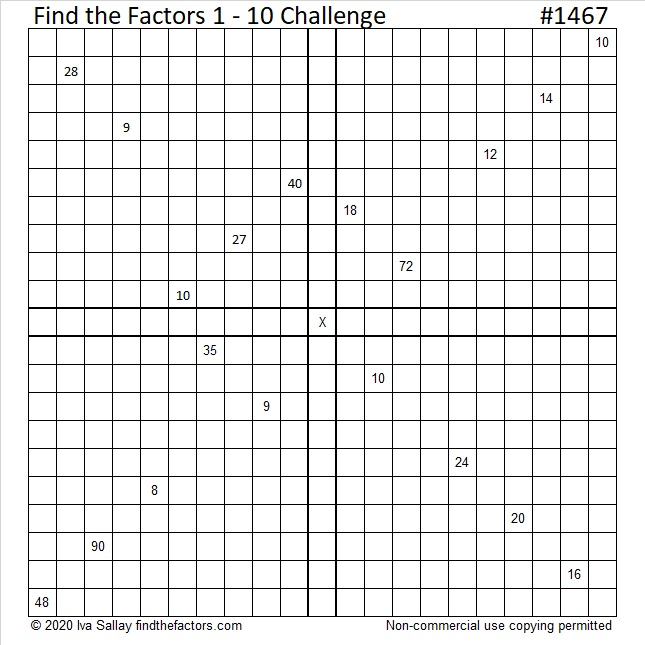Print the puzzles or type the solution in this excel file: 10 Factors 1454-1467

### Factors of 1467:

1467 is divisible by 3 because 1 + 4 = 5 and 5, 6, 7 are three consecutive numbers. Since the middle number, 6, is divisible by 3, we know that 1467 is also divisible by 9.

Of course, you could also add up the digits of 1467 to get 1 + 4 + 6 + 7 = 27, a number divisible by both 3 and 9, to know that 1467 is divisible by both 3 and 9.

• 1467 is a composite number.
• Prime factorization: 1467 = 3 × 3 × 163, which can be written 1467 = 3² × 163
• 1467 has at least one exponent greater than 1 in its prime factorization so √1467 can be simplified. Taking the factor pair from the factor pair table below with the largest square number factor, we get √1467 = (√9)(√163) = 3√163
• The exponents in the prime factorization are 2 and 1. Adding one to each exponent and multiplying we get (2 + 1)(1 + 1) = 3 × 2 = 6. Therefore 1467 has exactly 6 factors.
• The factors of 1467 are outlined with their factor pair partners in the graphic below.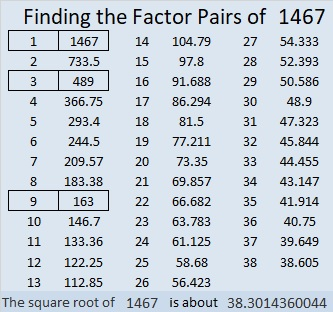# 695 and Level 1

• 695 is a composite number.
• Prime factorization: 695 = 5 x 139
• The exponents in the prime factorization are 1 and 1. Adding one to each and multiplying we get (1 + 1)(1 + 1) = 2 x 2 = 4. Therefore 695 has exactly 4 factors.
• Factors of 695: 1, 5, 139, 695
• Factor pairs: 695 = 1 x 695 or 5 x 139
• 695 has no square factors that allow its square root to be simplified. √695 ≈ 26.36285.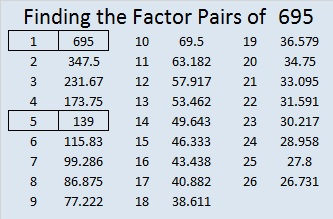Here is today’s puzzle: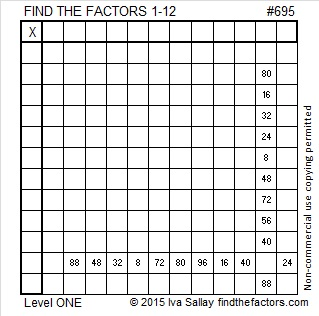Print the puzzles or type the solution on this excel file: 12 Factors 2015-11-30

———————————————————————————

Here are a few more facts about the number 695:

695 is the sum of the eighteen prime numbers from 7 to 73.

695 is also the hypotenuse of the Pythagorean triple 417-556-695. What is the greatest common factor of those three numbers?

137 + 138 + 139 + 140 + 141 = 695 so it is the sum of 5 consecutive numbers.

Since 695 is odd, it is the sum of two consecutive numbers, 347 and 348.

———————————————————————————# 671 is the Magic Sum of an 11 x 11 Magic Square

6 – 7 + 1 = 0 so 671 is divisible by 11.

671 is the sum of the fifteen prime numbers from 17 to 73.

Because 61 is one of its factors, 671 is the hypotenuse of the Pythagorean triple 121-660-671. The greatest common factor of those three numbers practically jumps out at me. Does it do the same thing to you?

Best of all 671 is the magic sum of an 11 x 11 magic square. (That link from wikipedia helped me construct this square. I’ll give directions so you can do it, too!)Notice how every row, column, and diagonal on the square sums to 671. The reason it is the magic sum is because the sum of all the numbers from 1 to 121 can be computed and then divided by 11 (the number of rows). Here is the equation:

• 671 = 121 x 120/2/11

Because 11 is an odd number there are simple directions to complete the entire square:

The number 1 is located in the exact center of the top row.

Find the number 2 on the square. (It’s located on the bottom row just right of the exact center square.) Notice that the numbers 3, 4, 5, and 6 are on the same diagonal. If you imagine the diagonal wrapping around the square, you can continue to follow it for numbers 7, 8, 9, 10, and 11. We can’t put the number 12 along the same diagonal because the number 1 is already in that spot, so we put the 12 UNDER the 11 and begin working on a new diagonal.

Anytime a number already occupies a space on a diagonal, put the next number under the preceding number and continue making a new diagonal. When a diagonal reaches the edge of the square, imagine that edge is connected to the opposite edge and continue the diagonal from the opposite edge.

I found it to be the trickiest placing the numbers 67 and 68, but other than that it was rather easy to know where to put the numbers.

Notice that the difference between any smaller number and the larger number just below it is either 12 or 1.

If you have excel on your computer, click on 12 Factors 2015-11-02, select the magic square tab, and then you can make this 11 x 11 magic square yourself. As you type in numbers, the columns, rows, and diagonals will automatically keep a running sum.

Once you get the square to give the magic sum in each direction, you can try doing the same thing with the 13 x 13 magic square that I’ve included on the same page. Its magic sum is 1105 which can be also be computed:

• 1105 = 169 x 170/22/13.

There is actually many more possible and probably more complicated 11 x 11 and 13 x 13 magic squares. I hope you enjoy making some with this easy method.

—————————————————————————————————

• 671 is a composite number.
• Prime factorization: 671 = 11 x 61
• The exponents in the prime factorization are 1 and 1. Adding one to each and multiplying we get (1 + 1)(1 + 1) = 2 x 2 = 4. Therefore 671 has exactly 4 factors.
• Factors of 671: 1, 11, 61, 671
• Factor pairs: 671 = 1 x 671 or 11 x 61
• 671 has no square factors that allow its square root to be simplified. √671 ≈ 25.903668.—————————————————————————————————

# 590 and Level 5

3(20^2)/2 – 20/2 = 600 – 10 = 590. That means that 590 is the 20th pentagonal number.

590 is also the hypotenuse of the Pythagorean triple 354-472-590. Which factor of 590 is the greatest common factor of those three numbers? Hint: In this case the GCF is the difference between the middle number in the triple and either of the other two numbers.Print the puzzles or type the solution on this excel file: 10 Factors 2015-08-17

—————————————————————————————————

• 590 is a composite number.
• Prime factorization: 590 = 2 x 5 x 59
• The exponents in the prime factorization are 1, 1, and 1. Adding one to each and multiplying we get (1 + 1)(1 + 1)(1 + 1) = 2 x 2 x 2 = 8. Therefore 590 has exactly 8 factors.
• Factors of 590: 1, 2, 5, 10, 59, 118, 295, 590
• Factor pairs: 590 = 1 x 590, 2 x 295, 5 x 118, or 10 x 59
• 590 has no square factors that allow its square root to be simplified. √590 ≈ 24.2899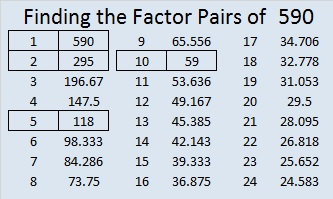—————————————————————————————————# 581 and Level 3

A year ago I wrote a short, but heartfelt post for World Elephant Day. I invite you to read it or any other article that makes you aware of what you can do to protect elephants from poachers.

581 is the sum of consecutive primes two different ways: 191 + 193 + 197 = 581, and the sum of all the prime numbers from 19 to 71 also is 581.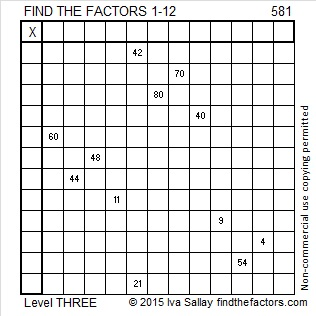Print the puzzles or type the solution on this excel file: 12 Factors 2015-08-10

—————————————————————————————————

• 581 is a composite number.
• Prime factorization: 581 = 7 x 83
• The exponents in the prime factorization are 1 and 1. Adding one to each and multiplying we get (1 + 1)(1 + 1) = 2 x 2 = 4. Therefore 581 has exactly 4 factors.
• Factors of 581: 1, 7, 83, 581
• Factor pairs: 581 = 1 x 581 or 7 x 83
• 581 has no square factors that allow its square root to be simplified. √581 ≈ 24.10394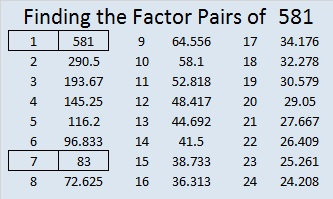—————————————————————————————————

A Logical Approach to solve a FIND THE FACTORS puzzle: Find the column or row with two clues and find their common factor. Write the corresponding factors in the factor column (1st column) and factor row (top row).  Because this is a level three puzzle, you have now written a factor at the top of the factor column. Continue to work from the top of the factor column to the bottom, finding factors and filling in the factor column and the factor row one cell at a time as you go.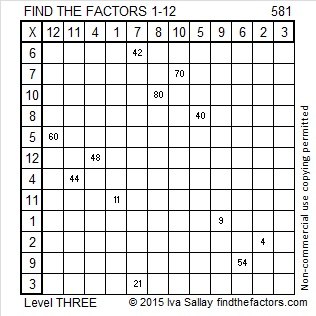# 580 and Level 2

580 is the sum of all the prime numbers from 83 to 107. Do you know what those consecutive prime numbers are?

580 is also the hypotenuse of four Pythagorean triples. In each case can you find the factor of 580 that is the greatest common factor of the triple?

• 68-576-580
• 96-572-580
• 348-464-580
• 400-420-580Print the puzzles or type the solution on this excel file: 12 Factors 2015-08-10

—————————————————————————————————

• 580 is a composite number.
• Prime factorization: 580 = 2 x 2 x 5 x 29, which can be written 580 = (2^2) x 5 x 29
• The exponents in the prime factorization are 2, 1, and 1. Adding one to each and multiplying we get (2 + 1)(1 + 1)(1 + 1) = 3 x 2 x 2 = 12. Therefore 580 has exactly 12 factors.
• Factors of 580: 1, 2, 4, 5, 10, 20, 29, 58, 116, 145, 290, 580
• Factor pairs: 580 = 1 x 580, 2 x 290, 4 x 145, 5 x 116, 10 x 58, or 20 x 29
• Taking the factor pair with the largest square number factor, we get √580 = (√4)(√145) = 2√145 ≈ 24.083189—————————————————————————————————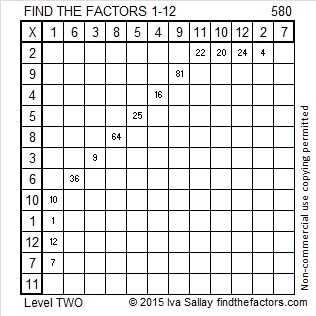# 579 and Level 1

579 is the hypotenuse of the Pythagorean triple 285-504-579. Which of 579’s factors is the greatest common factor of those three numbers?

Last week someone googled “find,the least 6 digit which has 173 factor” and arrived at Findthefactors.com. Here how to find the answer: Divide 100,000 by 173 and get 578.03 approximately. Round that answer up to 579. Multiply 579 by 173 and get 100167, the smallest 6-digit number that has 173 as a factor.Print the puzzles or type the solution on this excel file: 12 Factors 2015-08-10

—————————————————————————————————

• 579 is a composite number.
• Prime factorization: 579 = 3 x 193
• The exponents in the prime factorization are 1 and 1. Adding one to each and multiplying we get (1 + 1)(1 + 1) = 2 x 2 = 4. Therefore 579 has exactly 4 factors.
• Factors of 579: 1, 3, 193, 579
• Factor pairs: 579 = 1 x 579 or 3 x 193
• 579 has no square factors that allow its square root to be simplified. √579 ≈ 24.0624188—————————————————————————————————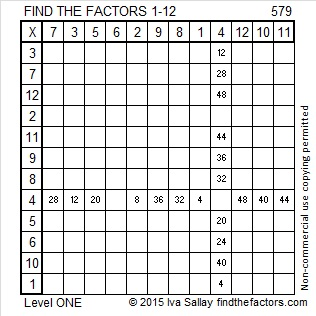# 572 and Level 1

72 can be evenly divided by 4 so 572 is divisible by 4.

5 – 7 + 2 = 0, which can be evenly divided by 11, so 11 is a factor of 572.

572 is the hypotenuse of the Pythagorean triple 220-528-572. Which factor of 572 is the greatest common factor of those three numbers?Print the puzzles or type the solution on this excel file: 10 Factors 2015-08-03

—————————————————————————————————

• 572 is a composite number.
• Prime factorization: 572 = 2 x 2 x 11 x 13, which can be written 572 = (2^2) x 11 x 13
• The exponents in the prime factorization are 2, 1, and 1. Adding one to each and multiplying we get (2 + 1)(1 + 1)(1 + 1) = 3 x 2 x 2 = 12. Therefore 572 has exactly 12 factors.
• Factors of 572: 1, 2, 4, 11, 13, 22, 26, 44, 52, 143, 286, 572
• Factor pairs: 572 = 1 x 572, 2 x 286, 4 x 143, 11 x 52, 13 x 44, or 22 x 26
• Taking the factor pair with the largest square number factor, we get √572 = (√4)(√143) = 2√143 ≈ 23.91652—————————————————————————————————# 564 and Level 6

Consecutive primes 281 and 283 add up to 564.

564 is made from three consecutive numbers so it can be evenly divided by 3. If the middle number is divisible by 3, then a number made from three consecutive numbers will also be divisible by 9. Is 564 divisible by 9? Why or why not?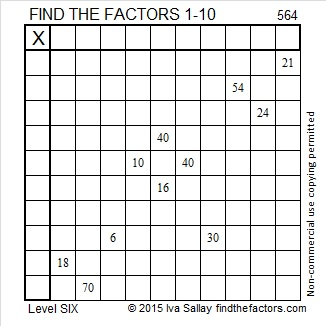Print the puzzles or type the solution on this excel file: 10 Factors 2015-07-20

—————————————————————————————————

• 564 is a composite number.
• Prime factorization: 564 = 2 x 2 x 3 x 47, which can be written 564 = (2^2) x 3 x 47
• The exponents in the prime factorization are 2, 1, and 1. Adding one to each and multiplying we get (2 + 1)(1 + 1)(1 + 1) = 3 x 2 x 2 = 12. Therefore 564 has exactly 12 factors.
• Factors of 564: 1, 2, 3, 4, 6, 12, 47, 94, 141, 188, 282, 564
• Factor pairs: 564 = 1 x 564, 2 x 282, 3 x 188, 4 x 141, 6 x 94, or 12 x 47
• Taking the factor pair with the largest square number factor, we get √564 = (√4)(√141) = 2√141 ≈ 23.74868—————————————————————————————————# 563 and Level 5

563 is prime, and it is the sum of all the prime numbers from 5 to 67.Print the puzzles or type the solution on this excel file: 10 Factors 2015-07-20

—————————————————————————————————

• 563 is a prime number.
• Prime factorization: 563 is prime.
• The exponent of prime number 563 is 1. Adding 1 to that exponent we get (1 + 1) = 2. Therefore 563 has exactly 2 factors.
• Factors of 563: 1, 563
• Factor pairs: 563 = 1 x 563
• 563 has no square factors that allow its square root to be simplified. √563 ≈ 23.727621How do we know that 563 is a prime number? If 563 were not a prime number, then it would be divisible by at least one prime number less than or equal to √563 ≈ 23.7. Since 563 cannot be divided evenly by 2, 3, 5, 7, 11, 13, 17, 19, or 23, we know that 563 is a prime number.

—————————————————————————————————